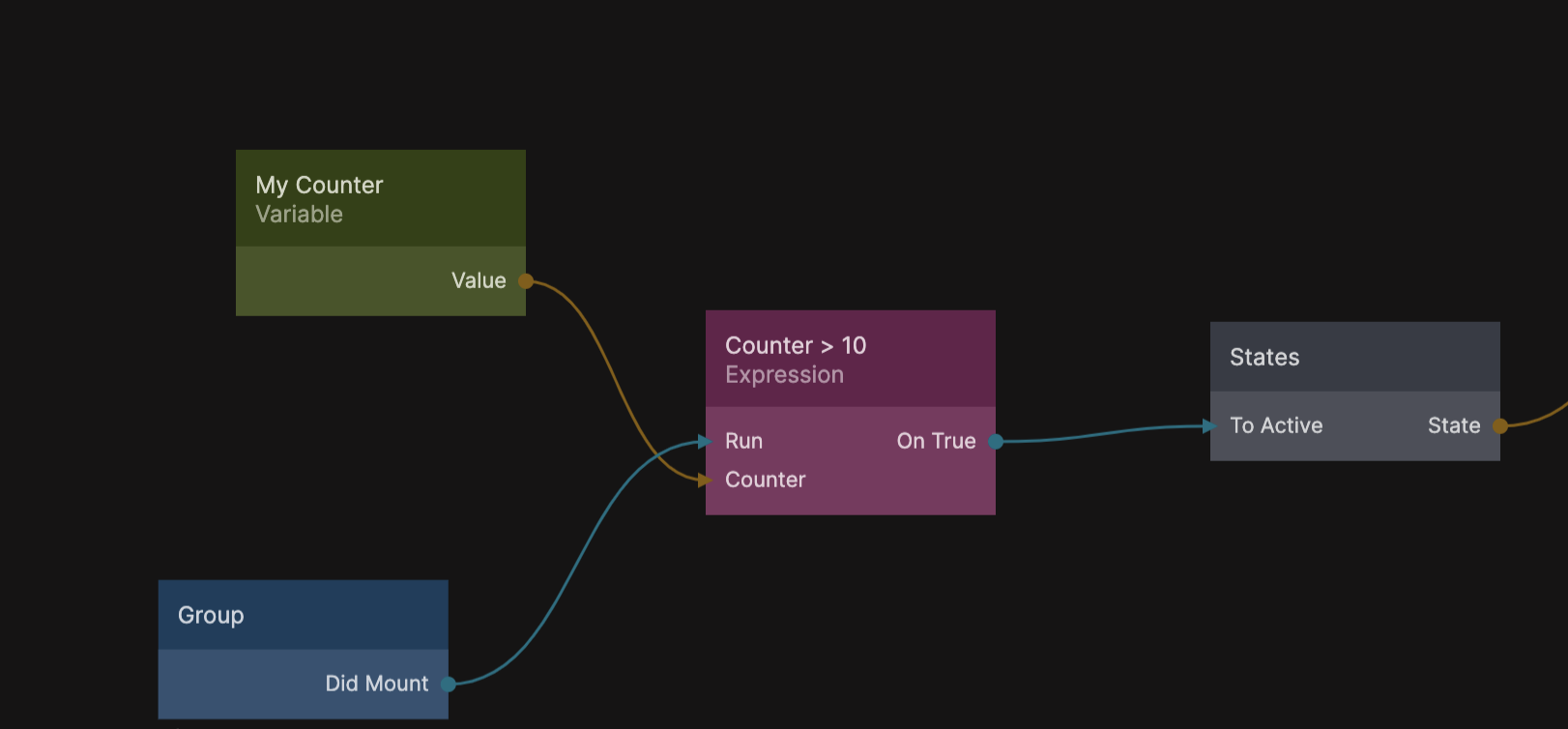# Expression

This node enables you to do your own calculations using simple expressons. Inputs are automatically derived from the expression. An expression set to `a + someName + 1` will get two inputs: one for a and one for someName.The Expression node runs JavaScript under the hood and supports a range of mathematical expressions.

## Built in math functions​

Expressions support the following funcitons:

• `min(a,b)` - Minimum of two values
• `max(a,b)` - Maximum of two values
• `cos(angle)` - The cosine of an angle in radians
• `sin(angle)` - The sine of an angle in radians
• `tan(angle)` - The tangent of an angle in radians
• `sqrt(a)` - Square root of a number
• `pi` - PI in radians
• `round(a)` - Rounds a number to the closest integer
• `floor(a)` - Removes the fractional part of a number
• `ceil(a)` - Rounds a value up to the closest higher integer. E.g. `round(1.1)` equals `2`.
• `abs(a)` - The absolute value of a number. Negative values will become positive.
• `random()` - A random number from 0 to 1

The Expression node is running JavaScript under the hood so in addition to the above functions any standard JavaScript functions will also be valid. The Expression node will add a `return` statement before the expression is executed. `1+2` will be evaluated as `return (1+2)`

## Truthy and Falsy signals​

You can use the signal outputs On True and On False to trigger actions based on the result of an expression, if the result is considered "truthy" or "falsy" in Javascript.

Normally, an expression node recalculates whenever one of the input parameters change. However, if you connect a signal to the Run signal input you can control when the expression is calculated. It will only calculate and update it's outputs when a signal is received.## Inputs​

ExpressionThe expression to be calculated.
RunNormally the expression is recalucated every time one of the input parameters change. But if you want to control when the expression is calculated you can connect a signal to this input, then the expression will only be calculated when a signal is received.

### Parameters​

Inputs are automatically derived from the expression. An expression set to `a + someName + 1` will get two inputs, one for `a` and one for `someName`.

As soon as any of the input values changes, the expression is reevaluated.